## Artigo

•Citado por SciELO
•Acessos

•Citado por Google
•Similares em SciELO
•Similares em Google

## versão impressa ISSN 0012-7353

### Dyna rev.fac.nac.minas vol.82 no.190 Medellín mar./abr. 2015

#### https://doi.org/10.15446/dyna.v82n190.43855

DOI: http://dx.doi.org/10.15446/dyna.v82n190.43855

A branch and bound hybrid algorithm with four deterministic heuristics for the resource constrained project scheduling problem (RCPSP)

Un algoritmo híbrido de ramificación y acotamiento con cuatro heurísticas determinísticas para el problema de programación de tareas con recursos restringidos (RCPSP)

Daniel Morillo-Torres a, Luis Fernando Moreno-Velásquez b & Francisco Javier Díaz-Serna b

a Universitat Politècnica de València, DSIC, Valencia, España. damotor3@posgrado.upv.es
b Facultad de Minas, Universidad Nacional de Colombia, Medellín, Colombia. lfmoreno@unal.edu.co, javidiaz@unal.edu.co

Received: June 4th, 2014. Received in revised form: September 9nd, 2014. Accepted: September 30th, 2014.

Abstract
This paper addresses the Resource Constrained Project Scheduling Problem (RCPSP). For its solution, a hybrid methodology, which uses a Branch and Bound basic algorithm with dominance rules, is developed and implemented, and is combined with four deterministic heuristics whose objective is to prune the search tree branches, taking into account the iterations available and, at the same time, to minimize the probability of discarding branches that contain optimal solutions. Essentially, these strategies allow the allocation of most iterations to the most promissory regions in an organized manner using only subsets with similar or the same characteristics as those of the optimal solutions at each level of the tree, thus assuring a broad search within the feasible region and, simultaneously, a good exploitation by the selective use of the subsets by level. Finally, the developed algorithm performance is analyzed by solving some of the problems of the PSPLIB test library.

Keywords: Project scheduling; resource constraints; deterministic heuristic methods; hybrid algorithm.

Resumen
En este artículo se aborda el problema de Programación de Tareas con Recursos Restringidos (RCPSP). Para su solución, se desarrolla y se implementa una metodología híbrida que usa como base un algoritmo de Ramificación y Acotamiento con potentes reglas de dominancia, y se combina con cuatro heurísticas determinísticas cuyo objetivo es truncar ramas del árbol de búsqueda, pero, a su vez, minimizar la probabilidad de descartar ramales que contengan soluciones óptimas. En esencia, estas estrategias permiten la repartición de iteraciones en forma mayoritaria y organizada en las regiones más promisorias usando, únicamente, subconjuntos que tengan características similares o iguales a las de las soluciones óptimas en cada nivel del árbol, garantizando así una amplia exploración dentro de la región factible y al mismo tiempo una buena explotación. Finalmente se analiza el desempeño del algoritmo desarrollado mediante la solución de algunos problemas de la librería de prueba PSPLIB.

Palabras clave: Programación de actividades; restricción de recursos; métodos heurísticos determinísticos; algoritmo híbrido.

1. Introduction

In operations research, there is a specific area of study known as Scheduling that aims to sequence a series of activities in time to execute them by means of an optimal allocation of resources, which are generally scarce. One of the most widely studied problems in this area is the Resource Constrained Project Scheduling Problem (RCPSP). Given the difficulty to find an optimal solution, but at the same time because of the need to find alternatives that are close to the optimum in reasonable time, several universities and organizations, especially software development companies, are working on it in many countries around the world.

Finding the solution to the RCPSP is still a challenge of great interest in the academic and business fields due to its high applicability, as it is one of the central problems in the planning, scheduling, and implementation of any project that may be divided into smaller units called tasks or activities, which usually are subject to two types of constraints: precedence and resources. The former indicate that each activity cannot be started before all its immediate predecessors have been completed. The latter indicate that the total resource consumption of the activities that are active at any time cannot exceed the availability of any of the resources, considered renewable, i.e., when some resource is released due to the completion of any of the active activities, this resource can be used for others. However, if there is sufficient availability of resources, they can be used to perform simultaneous activities.

Formally, the RCPSP can be defined as follows : It is given a project consisting of a set of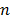activities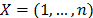, each of which requires, for its execution, a quantity of resources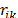whereis the activity andthe type of resource;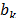is the total amount of resource typeavailable and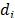the duration of the activity. Activities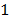andare fictitious, with zero duration and zero resource consumption, which represent the start and completion of the project .

Fig. 1 illustrates an example of the RCPSP taken from  that considers nine real and two fictitious activities, with three types of resources. The activity number is shown inside each node; the duration, at the top; and the consumption of each type of resource, at the bottom. Arrows indicate the precedence relations among the activities they connect; e.g., activity 3 is predecessor of 5 and 6, and successor of 1. It is assumed, without loss of generality, that activities are ordered in such a way that each predecessor activity ofis identified by a valuenumerically less than.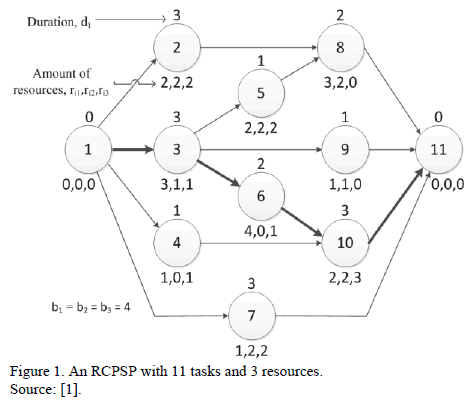The RCPSP solution is given by the start time of each one of the activities in such a way that the overall duration of the project or makespan is minimized. This combinatorial problem has been shown to be NP-hard [3,4]. Therefore, it is not feasible to solve medium or large instances by exact

algorithms, which guarantee to find the optimal solution. There are two approaches for the RCPSP solution: exact algorithms [1,5] and heuristic ones . New methods are permanently being designed, especially heuristic, attempting to find very close solutions to the optimal ones.

Regardless of the method used to find the RCPSP solution, it is important to calculate upper and lower bounds, which can be used as a stop criterion of the algorithm [7,8]. Relaxation models of the original problem are often used to find lower bounds. Therefore, if a solution for the original problem that is identical to a known lower bound is found somehow, it is known then that the optimal solution was reached. A lower bound used regularly is the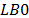, defined as the length of the longest route, which can be calculated using the Critical Path Method (CPM). This critical path appears in bold in Fig. 1. Although it may not be very useful for practical purposes, a simple way to calculate an upper bound for the makespan is using the following expression: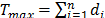, which considers sequential execution of all activities.

2. State of the art review

The origin of the RCPSP dates back to the late 50's of last century when intense research was carried out regarding the project planning, scheduling, evaluation, and monitoring in the area of operations research. As a result of these studies, CPM and PERT (Program Evaluation and Review Technique) were developed.

With these methodologies, more complex sequencing problems were tackled, which due to their combinatorial nature could not be solved in a reasonable time using traditional scheduling methods. As a result, some later studies were oriented to the development of heuristic algorithms for solving such types of problems.

Later on, the RCPSP was defined and in order to find its solution, several methodologies have been developed using traditional optimization as well as heuristic methods [6,9]. In , a first branch and bound algorithm based on the methodology proposed in  is presented. However, this algorithm can only solve small problems.

Subsequently, the efforts to solve the RCPSP were focused on the development of algorithms and methodologies that would determine the best lower and upper bounds in order to reduce the sample space and constraints. In , a lower bound based on Lagrangian simplifications of resource constraints is presented. In , lower bounds for the RCPSP based on the longest path of a model with minimization of the resource constraints are proposed. In  it is shown that Fisher's lower bounds and the like are better than those proposed by . Also, in , two new lower bounds for the RCPSP are proposed. The first one is based on a relaxation of the classical integer programming. The second one is based on a disjunctive graph obtained from the precedence network adding arcs that partially represent resource constraints.

In , a set of 110 problems of the RCPSP were generated and used as a starting point for benchmarking the algorithms developed to solve them. In , an exact branch and bound algorithm that significantly reduced the solution time of this set of 110 problems is presented.

The branch and bound approach combined with good lower bounds and appropriate dominance rules to remove the tree branches that do not contain the optimal solution is the best exact technique proposed for solving the RCPSP. In , three complexity indexes, Resource Factor (RF), Resource Strength (RS) and Complexity (C), which enable the RCPSP to be identified as "easy" or "difficult", are presented. RF measures the average number of resources used per activity. RS measures, for each resource, the relation between the available total resource and the average consumption of this resource; values close to 0 or 1 correspond to "easy" instances, and intermediate values correspond to difficult instances. The index C is a measure of network precedence complexity; the higher the value, the more complex the problem.

In , a new set of problems, called Project Scheduling Problem Library (PSPLIB), that serve to perform a new benchmark of the algorithms is proposed. Results show that the branch and bound approach proposed in  failed to solve these new sets of "difficult" problems in spite of the large computational time used.

In  a new mathematical formulation for the RCPSP is shown, based on the use of feasible subsets as decision variables, which had a good performance. In  a similar but more recent research is shown, using a new formulation defining a time horizon for each activity with new lower and upper bounds. Results achieved using CPLEX on PSPLIB library with a branch and bound scheme directly tailored for the new formulation are very good. In  an extensive study on the different new mathematical formulation is shown. Also, the event-based mixed integer linear programming is proposed.

Currently, research on the RCPSP focuses on finding practical solutions to the industry. So, heuristic methods that produce satisfactory results are used in order to find good approximations to optimal solutions using a relatively small computational effort .

Among the heuristics repeatedly mentioned in the literature are the population-based algorithms such as genetic and ant colony algorithms, which use random components as an escape strategy from local optimal solutions. In recent years, genetic algorithms have specialized in solving more complicated instances of the RCPSP, showing good results. Besides, there is a trend to use hybrid algorithms. For more information about this, refer to papers [22-29]. In  an experimental investigation of heuristics for the RCPSP is shown.

3. The proposed hybrid algorithm

This paper fills a gap identified in the literature between the performance of exact and heuristic algorithms in solving the RCPSP. A deeper analysis of the most advantageous features of the branch and bound is made, and an algorithm of this type is proposed as a basic method to guide a deterministic heuristic search.

This section consists of two sub-sections. In the first one, the branch and bound algorithm taken from the literature is clearly explained; then, a description of the four exact dominance rules used to prune the tree follows; some of these taken from the literature and others proposed by the authors in this paper.

The second sub-section contains the proposed heuristic, including the four heuristic strategies considered in the sequence generation scheme, the structure of the feasible solutions, and an illustration of the mechanisms, both exact and heuristic, incorporated in the algorithm to establish a balance between exploration and exploitation of the search in the feasible space. Finally three stopping criteria are shown.

3.1. Branch and bound algorithm

An algorithm of this type performs a tree-like exhaustive and organized search, creating branches that build the solution, from the root to an end node. From the literature review, it can be argued that among the exact algorithms, the branch and bound, presented in , is the best performing algorithm for the RCPSP, although it can be only useful for small instances. To streamline the search, the algorithm prunes some branches using exact dominance rules. Moreover, several different ways to improve the algorithm, which cut through dominance rules, have been developed.

The branch and bound algorithm operation in each of its stages is described below, differentiating the developments found in literature from contributions in this paper. For a better understanding some definitions are provided next. Level: the time at which a decision is made about which subset of activities is to be scheduled. Active task: a task that is executed in a time interval equal to its duration using the resources it requires. To release resources: to complete an active task, releasing the resources it requires. Eligible activity: an activity that satisfies all precedent constraints at a level. Power set of eligible activities: set of all subsets of eligible activities associated to a level, including the empty set and the set itself. Feasible subset: element of the power set that satisfies, along with the tasks that remain active, the resource constraints at a level; this element consists of eligible activities.

3.1.1. Steps of the algorithm

0) Initialization. The algorithm starts at level zero, at time zero. The number of iterations is determined.

1) Moving up to the next level. The algorithm determines the next time an activity is completed and releases a certain amount of resources, so that a subset of activities from the set of eligible activities can be scheduled. When the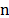activities of the project have been scheduled, a feasible solution is obtained, i.e., a complete sequence whose makespan value is used for comparing it with the best solution found so far, known as the incumbent solution.

2) Determining the set of eligible activities, the power set, sorted from larger to smaller number of activities, and the feasible subsets at a given level. In case of a tie, they are sorted according to the numbering of the activities. It is then evaluated which of these subsets are resource-based feasible, considering the activities or active tasks. Fig. 2 shows an example of the level 1 for the problem in Fig. 1.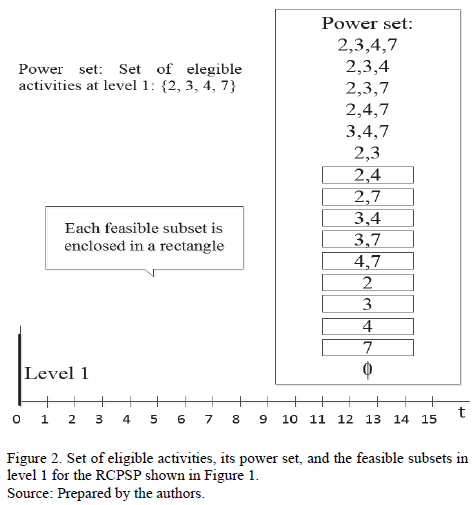At levels where there are no active tasks, e.g. level 1, it makes no sense to schedule the empty set, as this would only result in a delay in the overall project execution. However, it is possible to find the optimal sequence by scheduling the empty set at levels where there are active tasks. Nevertheless, in the set of test instances, the probability of this situation is very low.

3) Selecting the next feasible subset (per precedence and resources), for the current level in order to start its activities. If the level is explored for the first time, the first subset is chosen. When all the feasible subsets of the level have been scheduled, the algorithm moves to Step 4.

4) Backtracking down one level. The level and all activities scheduled from that time on are removed and the algorithm backtracks down one level. It is verified if the level reached is 0. If so, the algorithm is completed, otherwise, it returns to Step 3.

3.1.2. Dominance rules

Dominance rules are strategies based on mathematical reasoning developed to calculate exactly at which levels of the search tree it is no longer necessary to continue searching, as it is certain that a solution better than the incumbent one cannot be found. These rules enable regions of the feasible space where a search should no longer take place to be discarded, and thus allow greater efforts to be focused on performing iterations in other parts of the search space. Some of the dominance rules used in this paper, described below, were taken from the literature [13,14,16,31] and some others were prepared by the authors.

Rule 1: Precedence-based and resource-based critical path. It is used to truncate tree branches and can also be used as a stop rule. The precedence-based critical path is the well-known project critical path that can be obtained from a forward and backward review of the project network, calculating the early and late start of each activity. This estimation of the overall duration of the project only considers the structure of precedence, ignoring the resources.

The resource-based critical path (RCP) is a bound proposed by authors in this paper. It is calculated from a node to forward, only with activities that still remain to be scheduled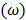based on the successor activities. Essentially, for each type of resource, the RCP is calculated with expression (1), choosing the longest duration. The minimum time the project would take to be completed is estimated if all activities were scheduled using all the available resources, i.e., with an efficiency of 100%.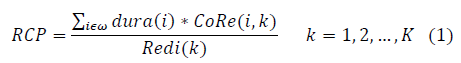Here,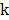represents the type of resource;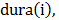the duration of activity i;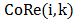, the consumption of resource type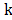by activity;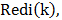the total available amount of the resource type. The critical path dominance is calculated from the precedence tree generated from each activity. The critical path by resources is calculated from the unscheduled activities in the partial solution. These two bounds can be calculated from any activity and are measured in time units. For any level, if the duration of the current partial solution plus the maximum between the RCP and the critical path is greater than or equal to the best stored time of a complete sequence, this branch of the tree should be pruned since a solution better than the current one will not be found. Thus, instead of scheduling the feasible subset of this level, the algorithm backtracks down one level.

Rule 2: Activity at a previous level. At each level, in step 3, when a feasible subset is to be scheduled, it is verified if any of its activities can be performed at a previous level. If so, this subset is discarded and the algorithm continues with the next subset. This is because if any activity of the feasible subset can be performed at a previous level, the resulting sequence would already have been scheduled and verified at that previous level because the branch and bound algorithm goes through the tree in a descending order according to the number of activities that can be scheduled. In this way, the partial solution being evaluated and all those that are generated from it cannot be better than the partial solution that considers the inclusion, at the current level, of any activity that could have been scheduled at a previous level. If the completion time of an activity of this type is greater than the time of the current level, the algorithm moves on to the next feasible subset. Otherwise, the entire level is discarded and the algorithm backtracks down one level.

Rule 3: Activity with the shortest duration at the same level. Fig. 3 shows an example of a diagram of this dominance, where the last scheduled activity was 3. The eligible activities are 4, 7 and 10 and the selected feasible subset contains activities 7 and 10. This dominance rule checks if the activity 4, that has the shortest duration, can be started along with activities 7 and 10. If so, this partial solution containing only the feasible subset 7 and 10 will be dominated.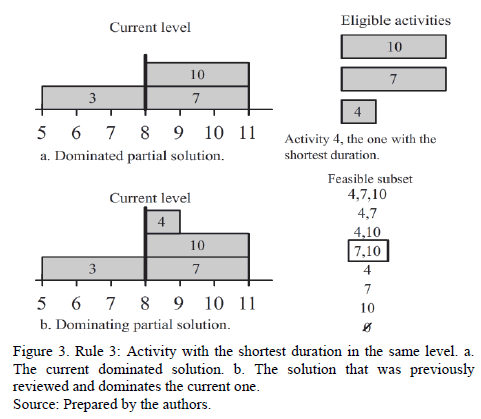Rule 4: Interchangeable activities. Successors of one activity are identified by a higher number. At each level, once a feasible subset is scheduled, the partial solution, i.e., the sequence of the scheduled activities, is analyzed, and it is verified if any activity can be interchanged with another scheduled one identified with a greater number, without violating the precedence and resource constraints. As this interchange can modify the start time of an entire set of activities, it is necessary to verify if the solution remains feasible. This test is performed for each pair of activities that do not share execution times and that, in the Gantt chart, the rightmost scheduled activity has a lower number than the activity to the left with which it is intended to be interchanged.

If a pair of activities can be interchanged, taking into account the previous conditions, the search tree should be pruned, i.e., backtracking down one level and removing that branch, since the algorithm must have already searched all sequences that can be generated from the current level in the interchanged sequence. After truncating a branch, the algorithm should move on to step 4.

In the literature reviewed, only two consecutive activities were interchanged. In this paper, we propose the general case where this verification is carried out with all previous candidate activities for exchange.

3.2. Proposed heuristic

Considering the branch and bound is the exact method that has given the best results , the algorithm base provides a sign of efficiency, since the branch and bound guarantees optimality. Although the hybrid heuristic proposed in this paper does not guarantee the optimal solution, it aims at reducing the computational time drastically, possibly giving up some accuracy regarding the optimum.

On the other hand, due to the way the algorithm is built, the search for sequences is carried out by considering only feasible solutions, using both precedence and resource constraints. This allows the heuristic not to worry about non-feasible solutions, unlike many other algorithms that must incorporate some mechanism to eliminate them or to penalize them.

Finally, the algorithm expansion using branches is made in such a way that in the search tree it never returns to a solution already considered. Therefore, the search is never at a standstill, i.e., it never remains stuck in a local optimal solution nor does it fall into repetitive cycles. This attribute will become an escape strategy from local optima, which is the essence of the proposed algorithm since, while it is searching at a very high level of the tree (far from the root), if it cannot find any better sequence and a larger search is desired, it can implement a rule to force itself to backtrack down one level (to get close to the root) in order to continue searching in new zones of the feasible region.

The proposed hybrid methodology, in this paper, incorporates four heuristic deterministic strategies that provide essential information and rules of movement, in order to achieve a guided and efficient search. Later, the best sequence found by the algorithm is adjusted by means of the Forward-Backward Improvement (FBI) method. A detailed explanation of each of the steps developed follows.

3.2.1. New sequence-generator scheme

A parallel sequence-generator scheme is based on the power set of the eligible activities. In order to schedule first those with the highest possibility of containing the optimal solution, at each level, this set of eligible activities is ordered in a descending way by three criteria described later on in the text. Each criterion is weighed with a weight calculated by experimentation, as detailed later, in heuristic 4. These criteria were chosen from the priority rules used to schedule activities , that in this research are extrapolated to be used in the scheduling of sets. After calculating such weights, the evaluation of each feasible subset is computed at each level according to the three criteria, to carry out later a descending ranking; in case of a tie, the set with the lowest activity number will be preferred.

Efficiency in the use of resources. It is assumed that the optimal sequence of any RCPSP instance should try to maximize the use of resources for each unit of time, trying to schedule activities in the most efficient way, assuming that the higher the number of resources, possibly the higher the number of activities scheduled simultaneously, and therefore, a shorter project makespan.

The total consumption of resources of each level is calculated by adding the consumption of each of its feasible subsets. Then, the proportion of resources used for each of these feasible subsets with respect to the total for each level is calculated.

To estimate the consumption of resources of each feasible subset it is assumed that there exists different types of resources and, therefore, for each one of these, a weighting is used to represent its importance, depending on how critical it is for the problem. The weighting of resource type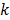is calculated by equation (2), where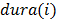is the time length of activity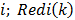is the total availability of resource type k; and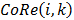, the use of resource type k in activity.Efficiency in releasing successor activities. The subsets of activities that release the largest number of successor activities are scheduled first, i.e., that after the first ones are finished, all the activities that had them as predecessors would satisfy the precedence constraints and would become eligible at the current level. At each level, after establishing the power set and verifying which the feasible subsets are, the total number of subsets that each feasible subset would release in the whole level is calculated, counting the repeated activities. Next, the proportion of activities released for each feasible subset is calculated, and results are ordered in a descending way according to this criterion.

Sets that minimize the project delay. This criterion, based on the CPM, considers the largest LFT (Late Finish Time) of all activities in the subset. An LFT associated to each feasible subset is calculated, adding the corresponding LFT of each one of its activities. In the same way, an LFT of reference for the level is obtained by adding the associated one of each of its feasible subsets. Next, the corresponding ratio of LFT for each feasible subset is calculated in relation to the total for the level, and results are ranked in ascending order according to such a ratio.

The purpose of this criterion is to schedule the lower LFT sets first to try to minimize the potential delay that may occur when an activity has been left out for later and scheduled at another level.

3.2.2. Allocation of iterations

The basic idea of the proposed heuristic is to guarantee exploration in all areas of the feasible region, by distributing the iterations throughout all branches. The systematic expansion is used as a strategy to avoid standstill in local optima. For instance, when half of the predetermined iterations have been executed, it would be expected that half of the feasible region has been searched, i.e., the algorithm is in the middle of the feasible subsets at each level. This does not mean that the search is performed in all the feasible subsets, but that these can be forced to change depending on the amount of executed iterations.

For each level, a counter of the number of iterations is restarted each time the algorithm moves up one level. This is because level 1 is actually the only one that keeps the total number of predetermined iterations for the algorithm. Each time that a feasible subset is scheduled at each level, the information related to the three criteria is updated, accumulating the amount of resources used, of successors released, and of minimal LFT's, which allows the update of the corresponding ratios applying heuristic 1 explained in subsection 3.2.1. The procedure used to implement this second heuristic, is synthesized in expression (3), valid for each level, as detailed next.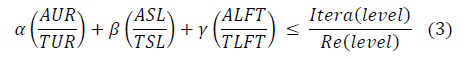The terms on the left of inequality (set relations) represent a weighted average of the three proportions, corresponding to the three criteria of heuristic 1, with weights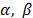and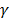, associated to each criterion, as defined later in heuristic 4. Numerators AUR, ASL, and ALFT, for the current subset, measure the cumulative of the use of resources, successors released, and LFT, respectively. Denominators TUR, TSL, and TLFT, for the level, measure the total of resources used, successors released, and LFT, respectively. The term on the right (iterations relation) measures the proportion between the number of iterations carried out, Itera, and the number of remaining iterations, Re.

If the member on the left is smaller than the term on the right, the scheduling of the corresponding level is delayed. Then, the algorithm cuts the tree at the highest level in which it is delayed to prevent stopping without exploring the whole feasible region, and to continue searching in the next feasible subset of that level. Depending on the way the feasible subsets are ordered and the set relations are computed, the hybrid algorithm makes the comparison regardless of the proportion of subsets run by level.

3.2.3. Pareto's principle

According to Pareto's principle, There are many causes or factors that contribute to the same effect, but only a small number contributes in a significant way to causing such an effect; that is, there are very few essential and many trivial causes; thus, a ratio of 80/20 is proposed, meaning that 80% of the effect is caused by 20% of the more important causes. To apply this principle, an experimentally computed proportion close to 60% (higher than 20% suggested by Pareto) of the eligible subsets is implemented at each level of the proposed algorithm. The computed proportion that varies depending on the problem complexity is calculated a priori for each case as shown below in strategy 4. The eligible subsets are ranked according to strategy 1, where the last ones on the list are the ones that have lower rank and therefore, have the least probability of containing the global optimum.

Self-adaptive parameters. Self-adaptive parameters are based on results of each of the iterations. As the branch and bound algorithm explores uniformly all the branches of the tree it is not possible, a priori, to obtain those branches that will be pruned or the number of solutions that will not be necessary to build, since they correspond to sequences dominated by some of the rules previously explained.

A heuristic pruning of branches can lead to the use of a smaller number of iterations than the one defined initially, ignoring thus part of the search in regions where better solutions could be found. To face this problem, a strategy is implemented so that after the branch and bound heuristic algorithm finishes, it verifies if the number of iterations executed is lower than the number established previously; if so, the algorithm restarts but searches only in regions within the feasible space with the pending iterations from the previous run.

Adaptive parameters. Adaptive parameters are based on a priori information about each problem, taking specific values in order to improve the algorithm operation. The proposed heuristic algorithm in this paper uses four parameters, three of which intervene in expression (3), as the weights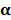,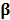and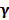. The fourth parameter is the Pareto's ratio of the selected subsets.

The goal of this heuristic is to use initial information about the problem to estimate, a priori, an indicator of the instance complexity, in order to adapt the values of the parameters, implementing a more aggressive strategy depending on how high the detected complexity or difficulty can be. Next the method of ranking of the instances and the complexity indices used are defined.

In the process of parameter adaptation, in the proposed heuristic, the instances of the problem are sorted first, for which an analysis was made of the academic library PSPLIB (Project Scheduling Problem Library), , specializing in sequencing problems, as described in section 4.

Of the complexity indices known in the literature, the inverse of the resources strength was chosen, defined as the average consumption of resources typeof all the activities divided by its total availability,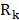, according to (4), where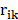is the consumption of resource typeby activity, and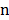is the total number of activities. To compare the various instances of the problem, this value was averaged with the values computed for all the types of resources, defining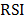(Resource Strength Inverse), as shown in (5).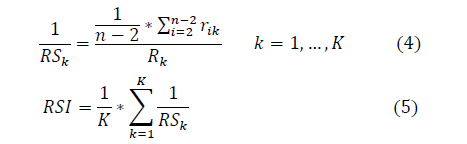The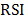for the 480 problems of set j30 was calculated and each value obtained was matched with the computing time obtained by . Results are shown in Fig. 4, where each point represents one of the 480 problems. It can be seen that in some cases, but not in others, as theapproaches 0.3, the computing time grows explosively; that is, if the computing time is high, anclose to 0.3 will be found, but a problem with anclose to 0.3 does not necessarily entail a high computing time. However, only a few cases do not satisfy this condition.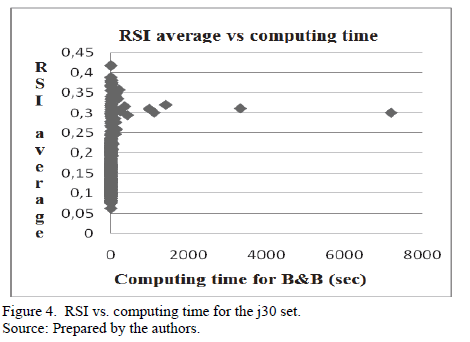RSI values for which it was considered that a RCPSP instance has a high level of difficulty were computed experimentally, giving a range between 0.259 and 0.355. Instances with RSI values outside this range can be associated with a low complexity level. Then, also experimentally, the best values of the four parameters,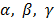, and the Pareto's proportion were determined, for both difficult and easy instances. Results are shown in Table 1.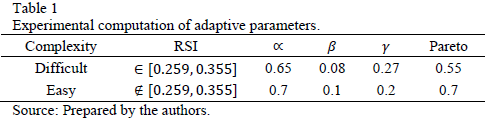Finally, the FBI method, , also known as double justification, is applied to the solution found. This method can be used as a complement of any other meta-heuristic since it is designed to improve a feasible solution previously found, using another algorithm. It is based on the backward/forward free space between activities, understood as its free time, in which it can be moved forward or backward, several times, with the purpose of scheduling the activities in the boundaries of their slack time, obtaining, in many cases, a decrease of the makespan.

This FBI method consists in executing at least one backward and one forward pass. In the first one, all the activities are sorted in descending order regarding their completion time; then a serial schedule generation scheme is developed, in such a way that all activities are shifted to the right at the latest time (late finish). In the forward pass, the activities are sorted in ascending order regarding their start time and a serial schedule generation scheme is applied in such a way that all activities are shifted to the left allowing them to start at the earliest time (early start), as much as their space permits it. Finally, it is verified if there was a better completion time for the project.

3.2.5. Three stopping criteria in the proposed hybrid algorithm

A previously defined limit of iterations. An iteration counts both when a complete sequence is established, i.e.,

when all the activities have a scheduled start time, and when using a dominance rule, it is determined that a partial sequence cannot be better than the current best solution.

A lower bound. The algorithm stops if it reaches a theoretical lower bound, as it is known that no solution can be better than such a bound. In this hybrid algorithm the lower bound of Stinson,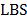, is used as a stopping criteria. It is described in .

Limit per level. The proposed hybrid algorithm distributes the iterations and continues the search depending on the proposed heuristic strategies. At each level, when all feasible subsets have been scheduled, the algorithm backtracks down one level, according to step 4, and the next feasible subset of the previous level is scheduled; if it has no next feasible subset, the algorithm backtracks down one level more. If there are no more subsets in all the higher levels, level zero is reached, where the algorithm stops because the entire feasible region has already been explored (not considering the truncated branches), using the predetermined iterations.

4. Test library and results

In  the PSPLIB library is proposed, which provides a set of problems for assessing the performance of the new heuristic schedule algorithms. It is available at: http://www.om-db.wi.tum.de/psplib/main.html.

Specifically for the RCPSP, there are four problem sets: j30, j60, j90 and j120 consisting of 30, 60, 90 and 120 activities, respectively. Each set has 480 problems except for j120 which has 600. In all sets, the same parameters were used to generate, and therefore they have all the difficulty levels.

In this section the set j30, consisting of 480 problems and 30 activities, was analyzed. The reason for this is that the problems in this set are the only ones where both the optimal solution and the solution time are known in an exact way and, therefore, comparisons with the algorithm developed in this research can be carried out without bias.

To evaluate the algorithm proposed efficiency, the 480 problems of the set j30 from the PSPLIB were solved. In order to validate the proposed algorithm, the percentage deviation of the found solution is calculated regarding the optimal solution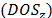for each instance, as shown in (6), where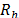is the solution obtained from the heuristic and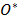is the instance optimal solution. In (7), the average of these deviations is calculated to obtain an average deviation of set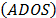. Table 2 shows a summary of the obtained results.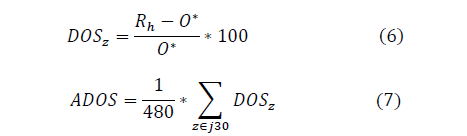In the first row, with 50,000 iterations, an average percentage deviation of 0.2436% is obtained with respect to the optimal solution, which is a highly satisfactory result.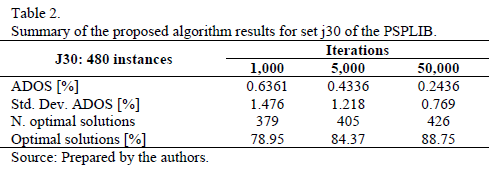Furthermore, as the number of the iterations increases, a lower percentage deviation is obtained, a foreseeable result that confirms the coherence of the algorithm, i.e., its proper operation.

The second row shows the standard deviation of this percentage deviation, which represents the error dispersion. In this case, deviations are small, indicating that their values are consistent and homogeneous, with little variability. Therefore, it can be concluded that the algorithm finds good results at different levels of difficulty. The third row shows the number of instances in which the proposed hybrid algorithm reaches the optimal solution. And the fourth row shows the corresponding percentage on the total of 480 instances.

Results of the proposed heuristic are comparable to ones obtained by some of the best meta-heuristic methods reported in the literature. A summary of results from these algorithms taken from  is shown in Table 3, where it can be seen that this research ranks number 19, being the only one in the list that uses a branch and bound as a basic algorithm and it is the only entirely deterministic heuristic algorithm of the list.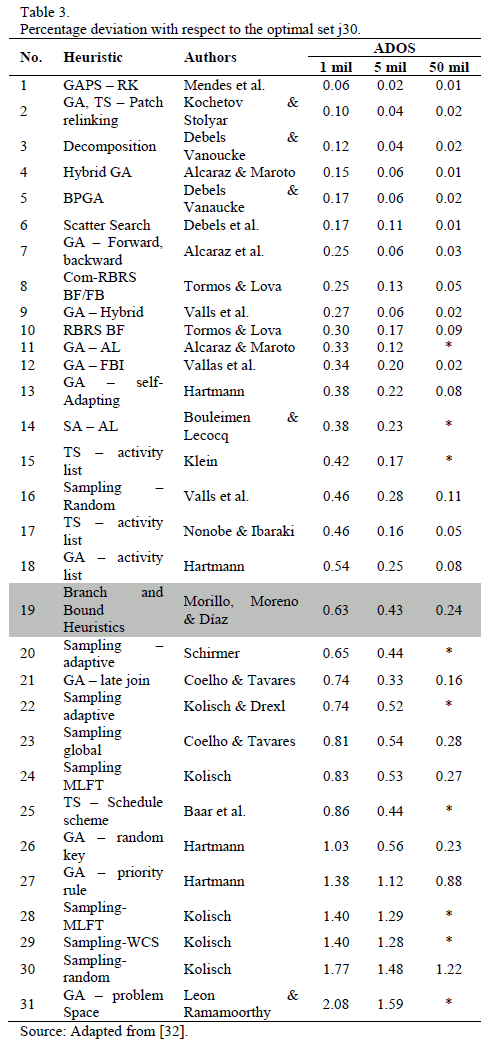Computational complexity. The exact Branch and Bound algorithm incorporates dominance rules and, in the worst case, it should go throughout the whole feasible solution space of the RCPSP. It is well known that this problem has an NP-hard complexity . However, the hybrid algorithm presented in this work, like, in general, all heuristics, greatly reduces the computational execution time, because the process is limited to the number of iterations chosen by the analyst, leaving subsequently as another component of complexity the own features of the method to construct feasible solutions .

In the proposed algorithm, these methods can be summarized as the use of SGS in parallel whose complexity order is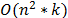and the ordering of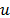eligible subsets at each level, with a complexity of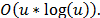5. Conclusions

There are several exact methods for the solution of the RCPSP. It is worth mentioning that the best is the Branch and Bound method because it solves the problem ensuring optimality. However, because it requires an extremely large computational effort, it is not applicable for high level complexity problems. The efficient alternative proposed in this paper is to solve the RCPSP by means of a hybrid algorithm integrating four deterministic heuristics into the branch and bound that capitalize on the advantageous characteristics of the exact algorithm but with the efficiency of the heuristic.

The reason for using the branch and bound as a base algorithm for formulating the hybrid heuristic lies not only on the attributes that make it attractive, but also on the missing of papers observed in the literature since this approach is not widely studied, in spite of its advantages. Thus, the purpose of this paper is to tackle the scarcity of reports related with the combination between exact algorithms, like the branch and bound, and heuristic algorithms for solving the RCPSP.

The proposed branch and bound heuristic hybrid algorithm uses the scheme of branch expansion proper to branch and bound, but built with the best sets at each time level where some set of activities can be scheduled (heuristics 1) but it does not tackle all the sets, only those with a higher probability of containing the optimum (heuristics 3). Besides, the tree is pruned at each level so as to force a search in the whole feasible space, according to the available iterations in a deterministic manner, this being the most important phase of the algorithm because it is the one that allows the escape from local optima (heuristics 2). In the same way, the parameters to obtain a better performance are adjusted using the information from each problem (heuristic 4). Finally, an FBI improvement is made to the solution obtained.

Results show that solutions can be obtained that are competitive with alternative algorithms commonly used for solving the RCPSP. The hybrid algorithm proposed in this research uses a basic exact algorithm, incorporating heuristic deterministic rules in order to increase its efficiency. Due to its favorable results, this approach could be considered as a starting point for future work along this research line.

References

 Mingozzi, A. et al., An exact algorithm for the resource constrained project scheduling problem based on a new mathematical formulation, Manage. Sci., 44 (5), pp. 714-729, 1995. http://dx.doi.org/10.1287/mnsc.44.5.714        [ Links ]

 Brucker, P. et al., Resourceconstrained project scheduling: Notation, classification, models and methods, Eur. J. Oper. Res., 112 (1), pp. 3-41, 1999. http://dx.doi.org/10.1016/S0377-2217(98)00204-5        [ Links ]

 Blazewicz, J., Lenstra, J.K. and Rinooy, K., Scheduling subject to resource constraints: classification and complexity, Discret. Appl. Math. - DAM, 5 (1), pp. 11-24, 1983.         [ Links ]

 Schäffter, M.W., Scheduling with forbidden sets, Discret. Appl. Math. [Online]. 72 (1-2), pp. 155-166, 1997. [date of reference March 06th of 2013]. Available at: http://dx.doi.org/10.1016/S0166-218X(96)00042-X http://dx.doi.org/10.1016/S0166-218X(96)00042-X        [ Links ]

 Morillo, D., Moreno, L. and Díaz, J., Metodologías analíticas y heurísticas para la solución del problema de programación de tareas con recursos restringidos (RCPSP): Una revisión Parte 1, Ing. y Cienc. [Online]. 10 (19), pp. 247-271, 2014. [date of reference April 24th of 2014]. Available at: http://publicaciones.eafit.edu.co/index.php/ingciencia/article/view/1982        [ Links ]

 Herroelen, W., De Reyck, B. and Demeulemeester, E., Resource-constrained project scheduling: A survey of recent developments, Comput. Oper. Res [Online]. 25 (4), pp. 279-302, 1998. [date of reference March 06th of 2013]. Available at: http://citeseerx.ist.psu.edu/viewdoc/summary?doi=10.1.1.95.1904        [ Links ]

 Brucker, P. and Knust, S., Lower bounds for resource-constrained project scheduling problems, Eur. J. Oper. Res., 149 (2), pp. 302-313, 2003. http://dx.doi.org/10.1016/S0377-2217(02)00762-2        [ Links ]

 Elmaghraby, S.E., Activity networks: Project planning and control by network models. John Wiley & Sons Inc, 1977.         [ Links ]

 Kolisch, R. and Padman, R., An integrated survey of deterministic project scheduling, Omega [Online]. 29 (3), pp. 249-272, 2001. Available at: http://linkinghub.elsevier.com/retrieve/pii/S0305048300000463 http://dx.doi.org/10.1016/S0305-0483(00)00046-3        [ Links ]

 Davis, E.W. and Heidorn, G.E., An algorithm for optimal project scheduling under multiple resource constraints, Manage. Sci., 17 (12), pp. B803-B816, 1 http://dx.doi.org/10.1287/mnsc.17.12.B803        [ Links ]

 Gutjahr, A.L. and Nemhauser, G.L., An algorithm for the line balancing problem, Manage. Sci., 11 (2), pp. 308-315, 1964. http://dx.doi.org/10.1287/mnsc.11.2.308        [ Links ]

 Fisher, M., Optimal solution of scheduling problems using lagrange multipliers Part1, Oper. Res., 21 (5), pp. 1114-1127, 1973. http://dx.doi.org/10.1287/opre.21.5.1114        [ Links ]

 Stinson, J.P., Davis, E.W. and Khumawala, B.M., Multiple resource-constrained scheduling using branch and bound, AIIE Trans. [Online]. 10 (3), pp. 252-259, 1978. [date of reference March 06th of 2013]. Available at: http://www.tandfonline.com/doi/abs/10.1080/05695557808975212        [ Links ]

 Christofides, N., Alvarez-Valdes, R. and Tamarit, J.M., Project scheduling with resource constraints: A branch and bound approach, Eur. J. Oper. Res. [Online]. 29 (3), pp. 262-273, 1987. [date of reference March 05th of 2013]. Available at: http://ideas.repec.org/a/eee/ejores/v29y1987i3p262-273.html        [ Links ]

 Patterson, J.H., A comparison of exact approaches for solving the multiple constrained resource, Project Scheduling Problem, Manage. Sci., 30 (7), pp. 854-867, 1984. http://dx.doi.org/10.1287/mnsc.30.7.854        [ Links ]

 Demeulemeester, E. and Herroelen, W., A branch-and-bound procedure for the multiple resource-constrained project scheduling problem, Manage. Sci. [Online]. 38 (12), pp. 1803-1818, 1992. [date of reference March 06th of 2013]. Available at: http://mansci.journal.informs.org/content/38/12/1803.full.pdf        [ Links ]

 Kolisch, R., Sprecher, A. and Drexl, A., Characterization and generation of a general class of resource-constrained project scheduling problems: Easy and hard instances, Res. Rep. No. 301, Inst. für Betriebswirtschaftslehre, Christ. zu Kiel.Germany., 1992.         [ Links ]

 Kolisch, R. and Sprecher, A., PSPLIB - A project scheduling library, Eur. J. Oper. Res., 96, pp. 205-216, 1996. http://dx.doi.org/10.1016/S0377-2217(96)00170-1        [ Links ]

 Bianco, L. and Caramia, M., A new formulation for the project scheduling problem under limited resources, Flex. Serv. Manuf. J. [Online]. 25 (1-2), pp. 6-24, 2011. [date of reference May 06th of 2014]. Available at: http://link.springer.com/10.1007/s10696-011-9127-y        [ Links ]

 Koné, O. et al., Event-based MILP models for resource-constrained project scheduling problems, Comput. Oper. Res. [Online]. 38 (1), pp. 3-13, 2011. [date of reference April 29th of 2014]. Available at: http://www.sciencedirect.com/science/article/pii/S0305054809003360        [ Links ]

 Hartmann, S. and Kolisch, R., Experimental evaluation of state-of-the-art heuristics for the resource-constrained project scheduling problem, Eur. J. Oper. Res., 127 (2), pp. 394-407, 2000. http://dx.doi.org/10.1016/S0377-2217(99)00485-3        [ Links ]

 Wang, H., Li, T. and Lin, D., Efficient genetic algorithm for resource-constrained project scheduling problem, Trans. Tianjin Univ. [Online]. 16 (5), pp. 376-382, 2010. [date of reference March 06th of 2013]. Available at: http://www.springerlink.com/index/10.1007/s12209-010-1495-y        [ Links ]

 Gonçalves, J.F., Resende, M. and Mendes, J., A biased random-key genetic algorithm with forward-backward improvement for the resource constrained project scheduling problem, J. Heuristics, 17 (5), pp. 1-20, 2011. http://dx.doi.org/10.1007/s10732-010-9142-2        [ Links ]

 Wang, X.-G. et al., Application of resource-constrained project scheduling with a critical chain method on organizational project management, in 2010 International Conference On Computer Design and Applications, 2, pp. V2-51-V2-54, 2010,         [ Links ]

 Elloumi, S. and Fortemps, P., A hybrid rank-based evolutionary algorithm applied to multi-mode resource-constrained project scheduling problem, Eur. J. Oper. Res. [Online]. 205 (1), pp. 31-41, 2010. [date of reference March 06th of 2013]. Available at: http://dx.doi.org/10.1016/j.ejor.2009.12.014 http://dx.doi.org/10.1016/j.ejor.2009.12.014        [ Links ]

 Montoya-Torres, J. R., Gutierrez-Franco, E. and Pirachicán-Mayorga, C., Project scheduling with limited resources using a genetic algorithm, Int. J. Proj. Manag., 28 (6), pp. 619-628, 2010. http://dx.doi.org/10.1016/j.ijproman.2009.10.003        [ Links ]

 Valls, V., Ballestin, F. and Quintanilla, S., A hybrid genetic algorithm for the resource-constrained project scheduling problem, Eur. J. Oper. Res., 185 (2), pp. 495-508, 2008. http://dx.doi.org/10.1016/j.ejor.2006.12.033        [ Links ]

 Deng, L., Lin, V. and Chen, M., Hybrid ant colony optimization for the resource-constrained project scheduling problem, J. Syst. Eng. Electron., 21 (1), pp. 67-71, 2010. http://dx.doi.org/10.3969/j.issn.1004-4132.2010.01.012        [ Links ]

 Feo, T.A. and Resende, M.G.C., A probabilistic heuristic for a computationally difficult set covering problem, Oper. Res. Lett., 8 (2), pp. 67-71, 1989. http://dx.doi.org/10.1016/0167-6377(89)90002-3        [ Links ]

 Kolisch, R. and Hartmann, S., Experimental investigation of heuristics for resource-constrained project scheduling: An update, Eur. J. Oper. Res. [Online]. 174 (1), pp. 23-37, 2006. [date of reference May 04th of 2014]. Available at: http://www.sciencedirect.com/science/article/pii/S0377221705002596        [ Links ]

 Brucker, P. et al., A branch and bound algorithm for the resource-constrained project scheduling problem, Eur. J. Oper. Res., 107 (2), pp. 272-288, 1998. http://dx.doi.org/10.1016/S0377-2217(97)00335-4        [ Links ]

 Cervantes, M., Nuevos métodos meta heurísticos para la asignación eficiente, optimizada y robusta de recursos limitados, Universidad Politécnica de Valencia, 2009.         [ Links ]

 Tormos, P. and Lova, A., A competitive heuristic solution technique for resource-constrained project scheduling, Ann. Oper. Res., 102 (1-4), pp. 65-81, 2001. http://dx.doi.org/10.1023/A:1010997814183        [ Links ]

D. Morillo-Torres, received a BSc in Industrial Engineering in 2010, and a MSc degree in Systems Engineering in 2013, both from the Universidad Nacional de Colombia, Medellin, Colombia. Currently, he is undertaking his PhD degree in Informatics at Universitat Politècnica de València, Valencia, España. His research interests include: mathematical modelling, operational research, heuristic optimization and scheduling. ORCID: 0000-0001-7731-1104

L.F. Moreno-Velásquez, received a BSc in Civil Engineering in 1973, a MSc degree in Mathematics in 1975, both from the Universidad Nacional de Colombia, Medellin, Colombia, and a MSc degree from Northwestern University at Evanston, Illinois, USA in 1979. From 1979 to 1993 he worked for several private companies. Since 1993 he has been working for the Universidad Nacional de Colombia as Associate Professor in the Computing and Decision Sciences Department, Facultad de Minas, Universidad Nacional de Colombia, Medellín, Colombia. His research interests include: optimization, operational research, heuristic optimization and scheduling and optimization tools.

F.J. Díaz-Serna, received a BSc in Industrial Engineering in 1982, a MSc degree in Systems Engineering in 1992, and a PhD degree in Systems in 2011, all of them from the Universidad Nacional de Colombia. Medellin, Colombia. Since 1983, he has worked as a Full Professor in the Computing and Decision Sciences Department, Facultad de Minas, Universidad Nacional de Colombia, Medellín, Colombia. His research interests include: optimization, simulation, and modeling in energy systems; nonlinear and mixed integer programming using computational techniques; and optimization tools. ORCID: 0000-0002-1849-8068